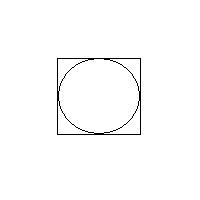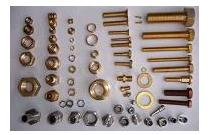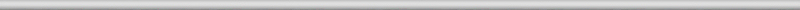## October 20, 2007

### Lengths of digital curves, continued

Filed under: computer vision/machine vision/AI, mathematics — Peter @ 4:24 pmRecall that in the last post we observed that, since a curve in a digital image is represented as sequences of points, it is natural to think of its length as the total sum of distances between consecutive points. However, with this approach the length of a diagonally oriented segment will be overestimated by 40%.

In the case of digital images, to compute the perimeter of an object we simply count the number of vertical and horizontal edges. Here is one interesting consequence. The perimeters of a square and the inscribed circle are the same!

Here is a more practical example. Suppose we want to classify objects in an image, like the one onthe right. Suppose we have detected and captured these objects and now all we want to do is to tell nuts from bolts. Let’s adopt a “dumb” approach and try to use the simplest method available. Then some of these objects can be classified based on size only. However, there are some bolts and nuts of about the same size and we have to look at their shapes. That does not work out as you’d expect.

The “roundness”, area/(perimeter squared), will be lower for elongated objects, like bolts. This works perfectly well in the continuous domain but in the digital domain it is possible to think of very different shapes with both area and perimeters exactly same. Take a diagonally oriented square with side a. Then its area is a*a and its (digital) perimeter is 4*√2*a. Now, a horizontally oriented rectangle (√2+1)*a by (√2-1)*a will have the same measurements. The proportions of this rectangle are about 1-to-5. It’s a bolt that looks like a nut

In the next post I will briefly discuss a paper on this subject.

### 3 Responses to “Lengths of digital curves, continued”

1. Computer Vision for Dummies » Lengths of digital curves, part 3 Says:

[…] Recall that in the previous posts we discussed what happens if one computes the length of a curve in a digital image as the total sum of distances between consecutive points. The conclusion was that using the length computed this way to evaluate the shapes of objects leads to disastrous results. […]

2. Computer Vision for Dummies » Lengths of digital curves, part 5 Says:

[…] Here is how it happened. The algorithm for roundness computes the perimeter first. More precisely, it computes the “adjusted” perimeter. The reason is that the perimeter computed as the number of steps in the curve depends on the orientation of the curve with respect to the grid. This “first degree approximation” produces the same roundness for a square and a 5×1 rectangle. To compute the better adjusted perimeter we use the “second degree approximation” that takes into account both the perimeter and the curvature (number of turns). Some error is still there but the results were supposed to be much better. And they were until I tried a diagonally oriented square. The roundness was way off! […]

3. Computer Vision for Dummies » Connectivity (or Lengths of Digital Curves, part 6) Says:

[…] Yes, part 6! I thought I was done with the topic (Part 1, Part 2, Part 3,…), but a couple of days ago I ran into this blog post: General connectivity (MATLAB Central) . The issue is connectivity in digital images: 4-connectivity, 8-connectivity, and other “connectivities”. The issue (adjacency of pixels) is discussed in the wiki. When I wrote this article though I did not realize that the topic is connected to measuring lengths of curves. Indeed, the 8-connectivity produces curves that go only horizontally or vertically while the 4-connectivity allows diagonal edges as well. In the post the curves appear as “perimeters” of objects. More accurately, they should be called contours or boundaries of objects as the perimeter is mathematically the length of the boundary (that’s where “meter” comes from). But bwperim is the name of the standard MATLAB command for finding the boundary and we will have to live with that… […]